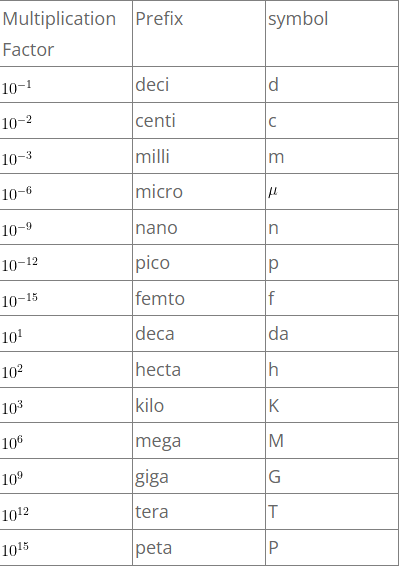# Special Units

Special Units

1. Length:

• Micron(mu )= 10^{-6}m = 10^{-4} cm
• Angstrom (A) = 10^{-10}m = 10^{-8}cm
• Fermi = 10^{-15}m = 10^{-13}cm
• Astronomical unit (A.U) = (1.5) 10^{11}m = (1.5) 10^{13}cm;
• What is 1 A.U. ? Ans: 1 A.U(Astronomical Unit) is the distance between Earth and Sun. 1A.U=(1.496) 10^{8}km.
• X ray unit (X.U) = 10^{-13}m (wave length of X-Rays)
• Light year = Distance traveled by light in one year= 9.5times 10^{15}m =9.5times 10^{12}km
• parsec = 3.26 light years = 3.1times 10^{16}m
• 1 mile = 1.609 km = 8 furlongs = 1760 yards = 5280 feet
• 1 agate(typography) = 0.07 inch = 1.8 centimeter
• 1 angstrom = 0.0000001 mm = 0.000000004 inch
• 1 cable’s length = 720 feet = 219.46 meters
• 1 chain (engineer’s) = 100 feet = 30.48 meters
• 1 chain (Gunter’s or surveyor’s) = 66 feet = 20.12 meters
• 1 cubit = 18 inches = 45.72 centimeter
• 1 degree (geographical) = 69.05 miles = 111.12 kilometers
• 1 decameter = 10 meters = 32.81 feet
• 1 fathom = 6 feet = 1.83 meters
• 1 hand = 4 inches = 10.16 centimeters
• 1 league = 3 miles = 4.83 kilometers
• 1 link (engineer’s) = 1 foot = 0.31 meter
• 1 link (Gunter's or surveyor’s ) = 7.92 inches = 10.16 centimeters
• 1 perch or pole = 16.5 feet = 5 meters
• 1 point (typography ) = 0.1 inch = 0.35 millimeter

2.Time :

• Solar day def: The time taken by earth to complete one rotation about its own axis with respect to sun is called solar day. (Average value for all the days of one year is Mean solar day).
• Sidereal day: It is 4.1min shorter than Mean solar day .
• sidereal year: 365.26 Mean solar day d) Solar year = 365.24 Mean solar day
• Lear year = The year in which February month has 29 days is called leap year. It is divisible by 4.
• Lunar month: Time taken by moon to complete one rotation around earth is lunar month = 27.3 days.

3. Mass:

• Atomic mass Unit ( a.m.u) : = frac{1}{12}of mass of C_{12} atom =frac{1}{12}timesfrac{12}{N} = frac{1}{6.023times10^{23}} gr = 1.67times10^{-24}gr = 1.67times10^{-27}kg.
• 1 assay ton = 29.167 grams = 1.03 ounces
• 1 carat = 200 milligrams = 3.09 grains
• 1 decigram = 100 milligrams = 1.54 grains
• 1 decagram = 10 grams = 0.35 ounce
• 1 pound = 0.45 kilograms

4. Pressure:

• Atmosphere =760 mmHg = 76times13.6times980 dyne/cm^{2} = 1.013times10^6 dyne/cm^{2} = 1.013times10^5 N/m^{2}or pa.
• Bar = 750 mmHg = 75times13.6times980 dyne/cm^{2}=10^6 dyne/cm^{2} = 10^5 N/m^{2}or pa.
• Torr =1 mm Hg =0.1times13.6times980 dyne/cm^{2}=1333 dyne/cm^{2}=133N/m^{2} or pa.

5. Area:

• Barn: this is unit of area, it is used to measure cross section of nuclei.
• Barn =10^{-28} m^{2}
• 1 acre = 4047 sq meters = 4840 sq yards = 43560 sq ft
• 1 are = 100 square meters = 1076.39 sq feet
• 1 square(building) = 100 sq feet = 9.29 sq meters
• 1 sq link = 62.73 sq inches = 404.69 sq cm
• 1 township = 36 sq miles = 93.24 sq kms

6. Wind speeds:

• light wind = 7 miles/hr
• light breeze = 11 miles/hr
• gentle breeze = 16 miles/hr
• moderate breeze = 20 miles/hr
• fresh breeze = 25 miles/hr
• strong breeze = 30 miles/hr
• moderate gale = 35 miles/hr
• fresh gale =45 miles/hr
• strong gale = 50 miles/hr
• whole gale = 60 miles/hr
• storm = 70 miles/hr
• hurricane = 80 miles/hr

7. Horse Power: It is the British Unit of power =746 w.

8. Special units of derived Physical Quantities:

S.N Physical Quantity C.G.S Unit S.I Unit
1 Force Dyne Newton
2 Work, energy Erg Joule
3 Power Watt
4 Pressure Pascal

Multiples, sub multiples of units

Multiples and sub multiples of units in S.I system:
Depending upon the magnitudes of physical quantities we measure, we have to use different multiplication factors suitable for that particular case. Here let us see some widely used multiplication factors.